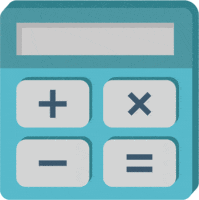# Math Worksheets Land

Math Worksheets For All Ages

# Math Worksheets Land

Math Worksheets For All Ages

Home > Tests >

# Grade 6 Math Tests and Quizzes

Sixth graders typical have a bigger learning curve as they transition into this math curriculum, more so than others. This is most likely because they need to be prepared to manage middle school math the following year. At this level, it is expected to have a solid foundation of arithmetic and the order of operations. Students previously were introduced to fractions, percentages, and decimals. These skills are drawn upon to help students understand the use and application of ratios and proportions. The big new concept at this level are unit rates. This topic is not only important for this course, but essential for science classes. Data and statistics have a large role in the monthly routine. Algebra starts to be more intensive as students are asked to write their expression based on math sentences. Having the notion that you should always combine like terms before attempting any algebra problem is a good one to have. I wrote all of the lower level tests before I wrote grade 6. Finally, I'm in my wheelhouse with the secondary level vocabulary. It would take me hours just to rewrite things for the level of vocabulary.

### What Topics Are Found on 6th Grade Math Tests?In most schools, students in the 6th grade are entering a middle school environment for the first time. They are giving a little more freedom and preparing the foundational skills that will be necessary to be a successful high school student. The 6th grade math curriculum introduces several completely new concepts, but for the most part extends spiral learning to the next level. There are five common areas of interest that students learn in the 6th grade. We will explore each of them for so that you will know how to prepare yourself or your students for a test at this level.

At this level ratios and proportions are usually taught at the start of the year. The main goal of that overall unit is to make sure that students understand a variety of methods for expressing a part of a whole value. They will learn to express this relationship as a ratio, proportion, percentage, decimal, fraction, and under the right circumstances unit rate.

Students also spend a good deal of time studying the number system with higher level operations and various number formats. Students are expected to be able to add, subtract, multiply, and divide decimals and fractions. The concept of factoring is explored in a much greater fashion at this level. You are expected to be able to find the greatest common factor and least common multiple of a factor pair. The coordinate plane makes an introduction to students, and they are expected to be able to plot a large number of ordered pairs and understand how to express the value of those points.

Prealgebra has an important role at this level. Students are expected to not only be able to understand expressions and equations but manipulate them and solve for missing variables. There is a great amount of time spent producing and evaluating equivalent expressions and equations. The concept of conditional expressions and inequalities is put in motion at this level. Students should know their way are both of those.

As usual geometry is short and sweet until we get to high school. The goal here is to use your knowledge of geometric figures to determine all types of measures. This includes nonuniform irregular area and perimeter. Students also learn how to pack or unpack these well-known shapes into nets.

We finish off the curriculum with a deluge of probability and statistics work. The focus seems to be on the measures of central tendency (mean, median, mode) and the application of them to everyday situations. Students spend a great deal of time understanding how to display data properly and decide if it valid. They eventually make their way to validating data distributions and identify explain the key measures in those data sets such as mean absolute deviation and interquartile tendencies.

Unlock all the answers, worksheets, homework, tests and more!
Save Tons of Time! Make My Life Easier Now

## Thanks and Don't Forget To Tell Your Friends!

I would appreciate everyone letting me know if you find any errors. I'm getting a little older these days and my eyes are going. Please contact me, to let me know. I'll fix it ASAP.